# Correlation coefficient

(diff) ← Older revision | Latest revision (diff) | Newer revision → (diff)

A numerical characteristic of the joint distribution of two random variables, expressing a relationship between them. The correlation coefficientfor random variables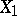and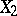with mathematical expectations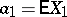and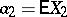and non-zero variancesand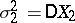is defined by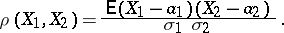The correlation coefficient ofandis simply the covariance of the normalized variables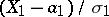and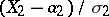. The correlation coefficient is symmetric with respect toandand is invariant under change of the origin and scaling. In all cases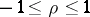. The importance of the correlation coefficient as one of the possible measures of dependence is determined by its following properties: 1) ifandare independent, then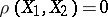(the converse is not necessarily true). Random variables for which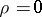are said to be non-correlated. 2)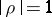if and only if the dependence between the random variables is linear: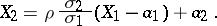The difficulty of interpretingas a measure of dependence is that the equalitymay be valid for both independent and dependent random variables; in the general case, a necessary and sufficient condition for independence is that the maximal correlation coefficient equals zero. Thus, the correlation coefficient does not exhaust all types of dependence between random variables and it is a measure of linear dependence only. The degree of this linear dependence is characterized as follows: The random variable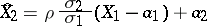gives a linear representation ofin terms ofwhich is best in the sense thatsee also Regression. As characteristic correlations between several random variables there are the partial correlation coefficient and the multiple-correlation coefficient. For methods for testing independence hypotheses and using correlation coefficients to study correlation, see Correlation (in statistics).

How to Cite This Entry:
Correlation coefficient. Encyclopedia of Mathematics. URL: http://encyclopediaofmath.org/index.php?title=Correlation_coefficient&oldid=12284
This article was adapted from an original article by A.V. Prokhorov (originator), which appeared in Encyclopedia of Mathematics - ISBN 1402006098. See original article